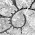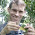## Tuesday, February 26, 2013

### On the shape of trees with random taxa addition or subtraction

Since I have a new function for add tips at random to a tree; and it is even easier to write a function to drop tips randomly from the tree - i.e., here it is:
drop.random<-function(tree,n=1)
tree<-drop.tip(tree,tip=sample(tree\$tip.label)[1:n])
I thought it might be fun to look at the effects of adding or subtracting tips at random from the tree. We already know that random missing taxa tends to create trees with longer than expected terminal edges - seemingly a slow-down in the rate of lineage diversification through time.

This is not very scientific, but first let's look at the LTT and Pybus & Harvey's (2000) γ for a single tree that we initiate with 100 species and then add to randomly using add.random in increments of 10. Here's our code (minus creating the video):
require(phytools)
tree<-pbtree(n=100,scale=1)
mas<-900
for(i in 1:(mas/10+1)){
x<-ltt(tree,plot=FALSE)
plot(x\$times[2:length(x\$times)],x\$ltt[2:length(x\$ltt)], xlab="time",ylab="lineages",log="y",type="l",xlim=c(0,1), ylim=c(2,max(x\$ltt)))
lines(c(0,1),c(2,max(x\$ltt)),lty=2)
text(x=0,y=max(x\$ltt),paste("N = ",length(tree\$tip), "\n","gamma = ",round(x\$gamma,3),sep=""),adj=c(0,1))
}

And here's the result:

OK, next, let's do the opposite - start with 1000 taxa and drop taxa random. First the code:
drop.random<-function(tree,n=1)    tree<-drop.tip(tree,tip=sample(tree\$tip.label)[1:n])
require(phytools)
tree<-pbtree(n=1000,scale=1)
menos<-900
for(i in 1:(menos/10+1)){
x<-ltt(tree,plot=FALSE)
plot(x\$times[2:length(x\$times)],x\$ltt[2:length(x\$ltt)],xlab="time", ylab="lineages",log="y",type="l",xlim=c(0,1), ylim=c(2,max(x\$ltt)))
lines(c(0,1),c(2,max(x\$ltt)),lty=2)
text(x=0,y=max(x\$ltt),paste("N = ",length(tree\$tip), "\n","gamma = ",round(x\$gamma,3),sep=""),adj=c(0,1))
tree<-drop.random(tree,n=10)
}

And the video:

Adding taxa at random, at least by our algorithm, does not seem to affect tree shape all that much; but subtracting random tips, as we expected, makes γ turn progressively more and more negative.

To see if this is idiosyncratic to the specific trees we started with, why don't we replicate the entire process (i.e., 900% addition or subtraction) in a single step for, say, 30 random pure-birth trees. Here's what that looks like. First, adding tips randomly:
XX<-matrix(NA,31,2,dimnames=list(NULL,c("gamma100","gamma1000")))
require(phytools)
for(i in 1:31){
tree<-pbtree(n=100)
XX[i,1]<-ltt(tree,plot=F)\$gamma
XX[i,2]<-ltt(tree,plot=F)\$gamma
}
colMeans(XX)
ZZ<-hist(XX[,2]-XX[,1],plot=F,breaks=-9.5:0.5)
barplot(ZZ\$density,names=ZZ\$mids,space=0,main=expression(gamma-gamma))

And the results:
> colMeans(XX)
gamma100  gamma1000
0.3568899 -1.9163830
And if we do the same thing dropping tips, here are the results:
> colMeans(YY)
gamma100  gamma1000
-6.1072618 0.2275565
So, on the face of it, it seems as though adding taxa randomly (from 100 to 1000 species in the tree) or dropping taxa (from 1000 to 100) both result in a decrease in γ - however the scale of decrease is highly asymmetrical, with random subtraction resulting in a much greater decrease in γ.

At the start of this little experiment I wasn't sure if random addition of taxa would increase or decrease γ, and in the end it seems to decrease γ but sometimes only a little.

1.I think the basis of what you're doing depends on the definition of 'at random'. In a previous post, you describe that you're adding tips as if the probability of an extra tip at any point along any edge increased with time. It isn't exactly clear, but I assume you let the probability increase linearly as branches approach the most terminal tips. This wouldn't be true under any birth/birth-death model; it's much more unlikely for a random tip to be attached further back in time than just a linearly decreasing probability. Just think of the exponential curve of a non-log-scaled LTT...

If I remember Nee et al. rightly, the probability of an additional random tip should be the same as the probability of having a branching time of time t, as that's just the probability of having a lineage which survived for duration t.

I think if you weighted tip addition in this way, relative to values of the birth and death rates, it would produce trees with no distortion of the estimated gamma parameter.

2.Actually, looking at your code, perhaps I misinterpreted your previous post about random new tips and cumsum. Now it looks like you're using it to pick an edge at random, with longer edges weighted to have a higher chance of getting chosen relative to their length? That's basically letting edges to go anywhere with uniform probability across the tree, something which would be unexpected under Yule/birth-death.

1.David - Yes, this is how the function works; and I agree that this should result in trees that are not Yule-like. - Liam

Note: due to the very large amount of spam, all comments are now automatically submitted for moderation.